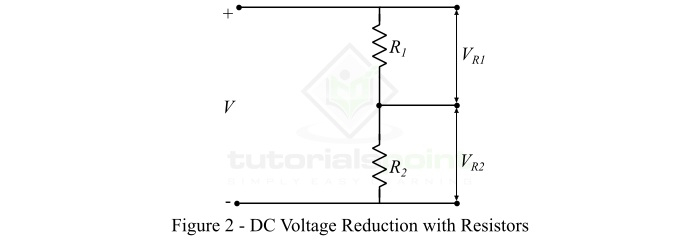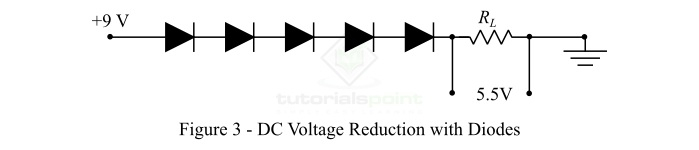# What is DC Voltage? – Definition, Circuit Symbol, and Wire Color Codes

In electrical and electronic circuits, the term voltagevoltage is used to denote the potential difference between two points. Based on the nature, the voltage can be of two types namely ŌĆō AC Voltage and DC Voltage.

In this article, we shall confine our attention to the DC Voltage only. Here, we will discuss the definition of DC voltage, its symbolic representation, DC wire color codes used in different electrical standards, and methods of DC voltage measurement. LetŌĆÖs begin with the basic definition of DC voltage.

## What is DC Voltage?

DC Voltage is an abbreviation of Direct Current Voltage. The voltage which has constant polarity, i.e. always forces a current through the circuit in one direction, is known as DC Voltage.

DC voltage may be a constant DC voltage or a variable DC voltage. A constant DC voltage is one whose magnitude and polarity both remain constant, while a variable DC voltage is one whose magnitude varies with time but the polarity remains constant.

DC voltage is generally obtained from a cell, battery, or a DC generator, etc. Since the polarity of a DC voltage does not change with the time, therefore, the DC voltage has zero frequency.

## DC Voltage Symbols

In electrical and electronic circuits, the symbol of dc voltage depends upon the type of source used. The symbols of a cell, a battery, and any dc voltage source are shown in figure-1.## DC Voltage Wire Color Codes

In electrical wiring, colors of wires are used to identify the polarity of the wire. Hence, the color coding makes the maintenance work easy to execute. There are mainly two color coding schemes used for DC wiring which are ŌĆō IEC DC Power Circuit Wire Color Codes and US DC Power Circuit Wire Color Codes.

### IEC DC Power Circuit Wire Color Codes

IEC stands for International Electrotechnical Commission. IEC defines a standard for DC power circuit wire color codes. The colors of different wires in a DC power circuit are listed in the following table ŌłÆ

DC System Wire Name & Label Wire Color
Ground or Earth PE Green-Yellow Strips
Two wire ungrounded DC circuit Positive "L+" Brown
Negative "L-" Grey
Two wire grounded DC circuit Positive (with negative grounded) circuit "L+" Brown
Negative (with negative grounded) circuit "M" Blue
Positive (with positive grounded) circuit "M" Blue
Negative (with positive grounded) circuit "L-" Grey
Three wire grounded DC circuit Positive "L+" Brown
Mid-Wire (Center tap) "M" Blue
Negative "L-" Grey

### US DC Power Circuit Wire Color Codes

Another DC voltage wire color coding system is US National Electrical Code. It is also called NEC (National Electrical Code). This system does not define the wire color codes for ungrounded DC systems because according to US National Electrical Code, the ungrounded DC system is unsafe to use. The wire color code recommendations for DC voltage system as per the US national electrical code are listed in the following table ŌłÆ

DC System Wire Name & Label Wire Color
Protective Ground or Earth PG Bare conductor or Green or Green-Yellow Strips
Two wire ungrounded DC system Positive "L+" No recommendation (Red is used).
Negative "L-" No recommendation (Black is used).
Two wire grounded DC system Positive (with negative grounded) circuit "L+" Red
Negative (with negative grounded) circuit "N" White
Positive (with positive grounded) circuit "N" White
Negative (with positive grounded) circuit "L-" Black
Three wire grounded DC system Positive "L+" Red
Mid-wire "N" White
Negative "L-" Black

## Methods of Reducing DC Voltage

Sometimes, we need to reduce the DC voltage before applying it across the load. For example, if we have a battery of 9 V, and an LED lamp of 3 V. In this case, we required to reduce the DC voltage so that it does not damage the LED. We can reduce the DC voltage using resistors and diodes.

### Reduction of DC Voltage using Resistors

When resistors are joined in series, they form a voltage divider circuit. The voltage divider circuit splits a single DC voltage into multiple DC voltages of lower values. Using resistors connected in series, we can get any desired voltage. A typical circuit for reducing the DC voltage is shown in Figure-2.In this circuit, V is the total input voltage. The voltages across the resistance ­Øæģ1 and ­Øæģ2 are determined by the following expressions ŌłÆ

$$\mathrm{V_{R1}=\frac{VR_{1}}{R_{1}+R_{2}}}$$

And,

$$\mathrm{V_{R2}=\frac{VR_{2}}{R_{1}+R_{2}}}$$

Hence, using these two equations, we can calculate the value of ­Øæģ1 and ­Øæģ2 to insert in the circuit to obtain the desired DC voltage.

### Reduction of DC Voltage using Diodes

A diode is a unidirectional electronic device that conducts only when forward biased. When a diode is forward biased, some voltage drop occurs in the diode. This voltage drop in a typical silicon diode is about 0.6 V to 0.7 V, and in germanium diode is approximately 0.25 V to 0.3 V. This voltage drop can further be increased by connecting a number of diodes in series with the load as shown in Figure-3.In the example shown in Figure-3, the voltage of a 9 V battery is reduced to approximately 5.5 V, and the rest 3.5 V (if each diode has 0.7 V drop) is dropped across the diodes.

## How to Increase DC Voltage?

The dc voltage is increased by using an electronic circuit known as DC-DC Power Converter or Boost Converter. A typical boost converter consists of at least two semiconductor switches either diode or transistor and one energy storing element either inductor or capacitor.

Note ŌłÆ There is an electronic circuit known as buck converter used to decreases the DC voltage.

## Measurement of DC Voltage

The following are some common method for the measurement of DC voltage ŌłÆ

• DC voltage can be measured using a DC voltmeter.

• We can also measure the DC voltage using a multimeter.

• Sometimes, OhmŌĆÖs law is also used to calculate the DC voltage, when we have the values of circuit current and resistance.

## Conclusion

In this article, we discussed all the important concepts related to the DC voltage. DC voltage is a constant polarity voltage that forces an electric current through the circuit to flow in one direction only. However, the magnitude of the DC voltage can be either constant or time varying. DC voltage is primarily used in electronic circuits. These days, a multimeter is most commonly used for the measurement of DC voltage due to its simplicity.